The puzzlehunt has ended, thanks for playing!

# Naughty Crosses

## Solution

solution: Daan van de Weem and Hannah Earley

We are presented with a grid and a title, 'Naughty Crosses', and the grid appears clickable. The title suggests that this might be a game of 'Noughts and Crosses', and indeed when we start clicking we find that the board is populated by X's (us) and O's (the computer). However, upon playing a few games we quickly find that the game is not as it seems and a standard horizontal, vertical or diagonal line does not result in a win for either us or the computer.

If we let the computer win, however, then we can begin to find the solutions: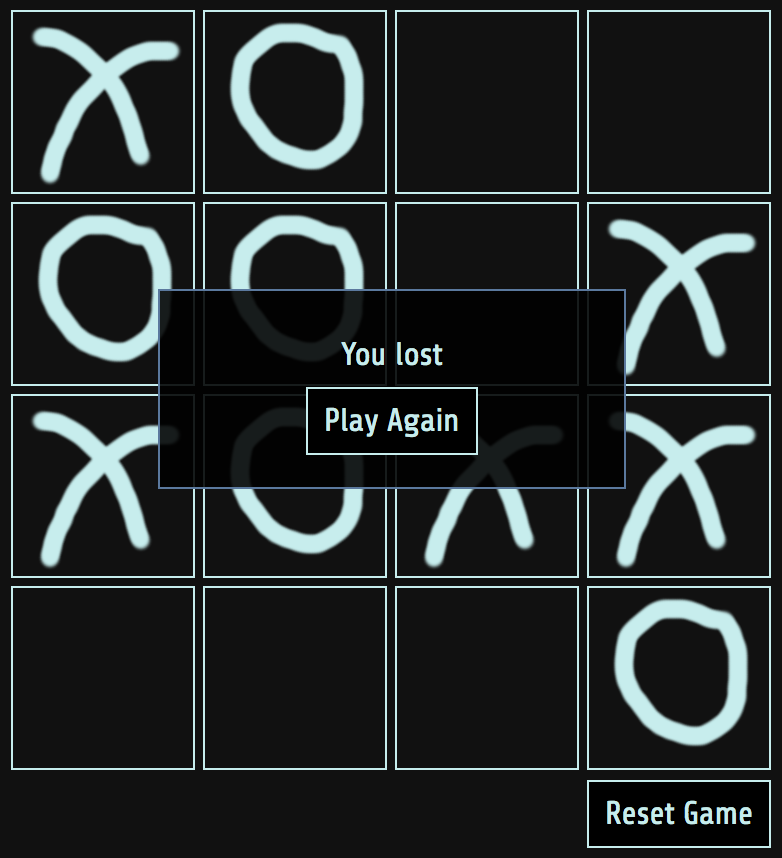If we then try to play the same strategies we can then find the winning solutions: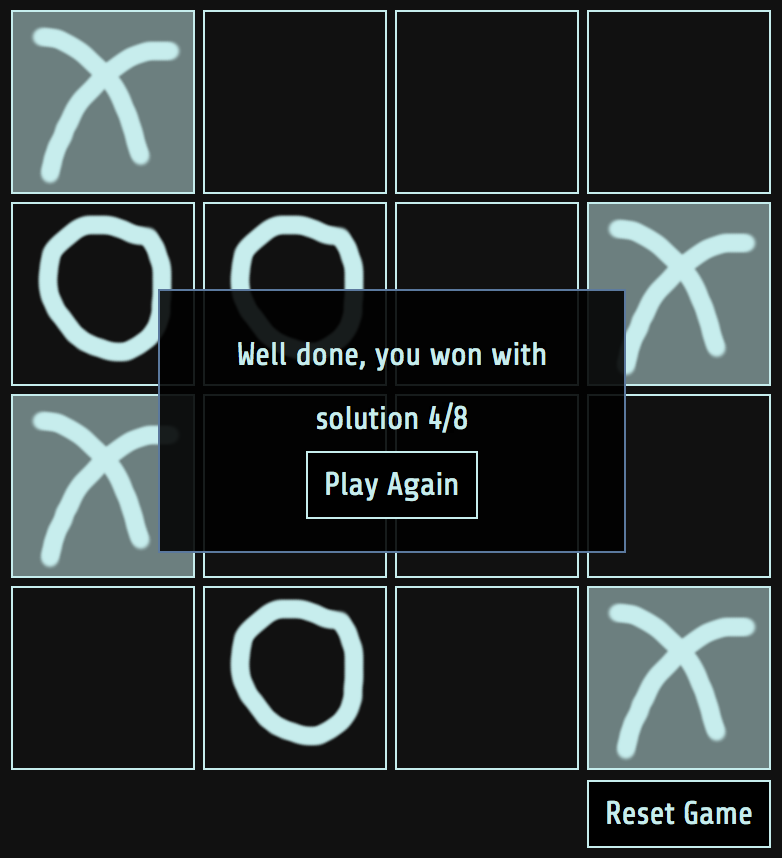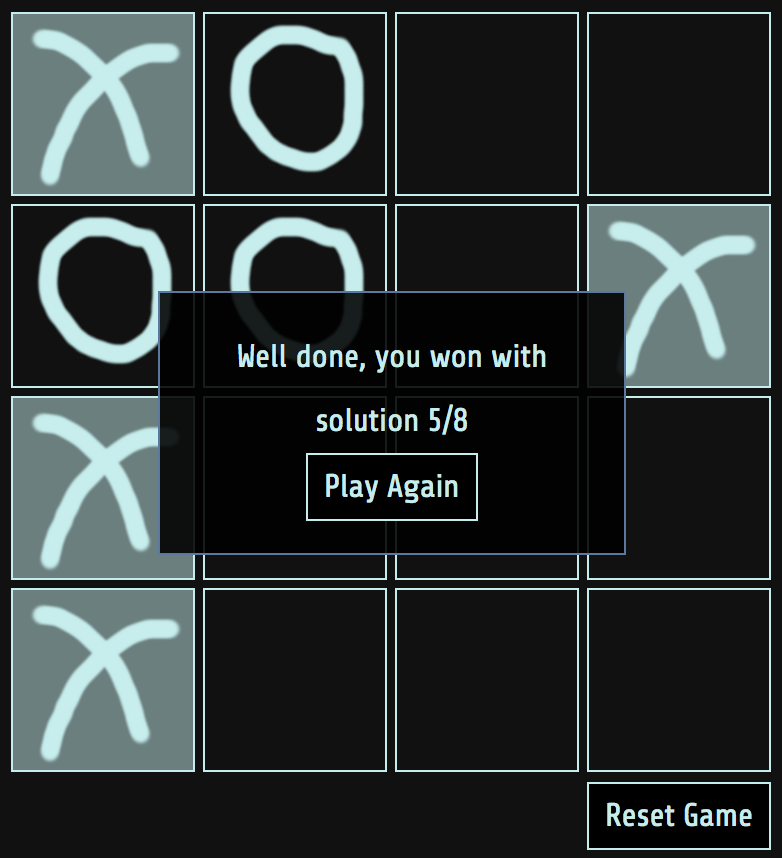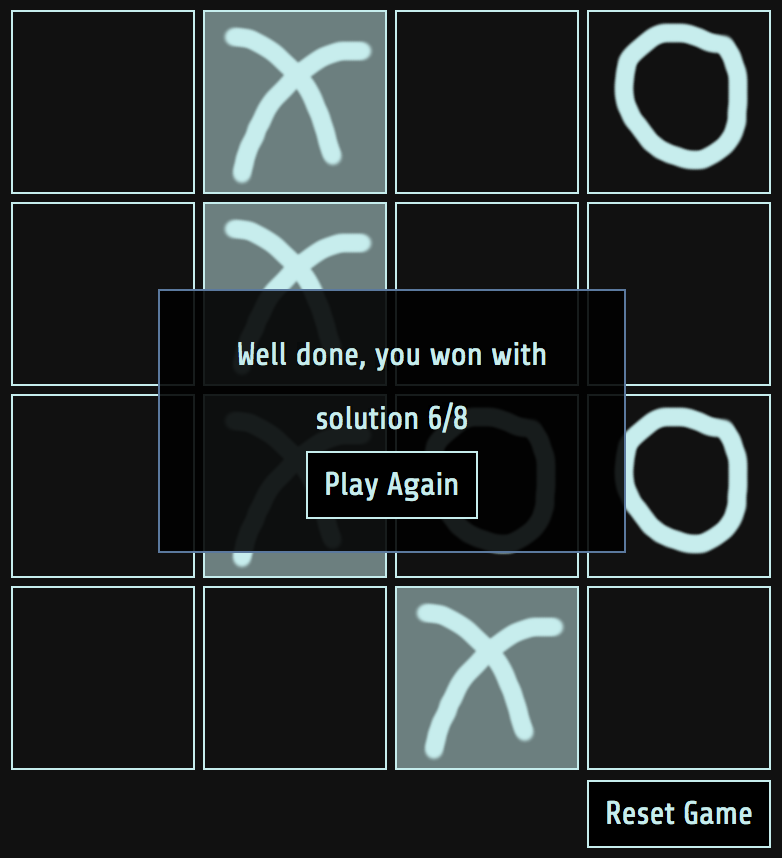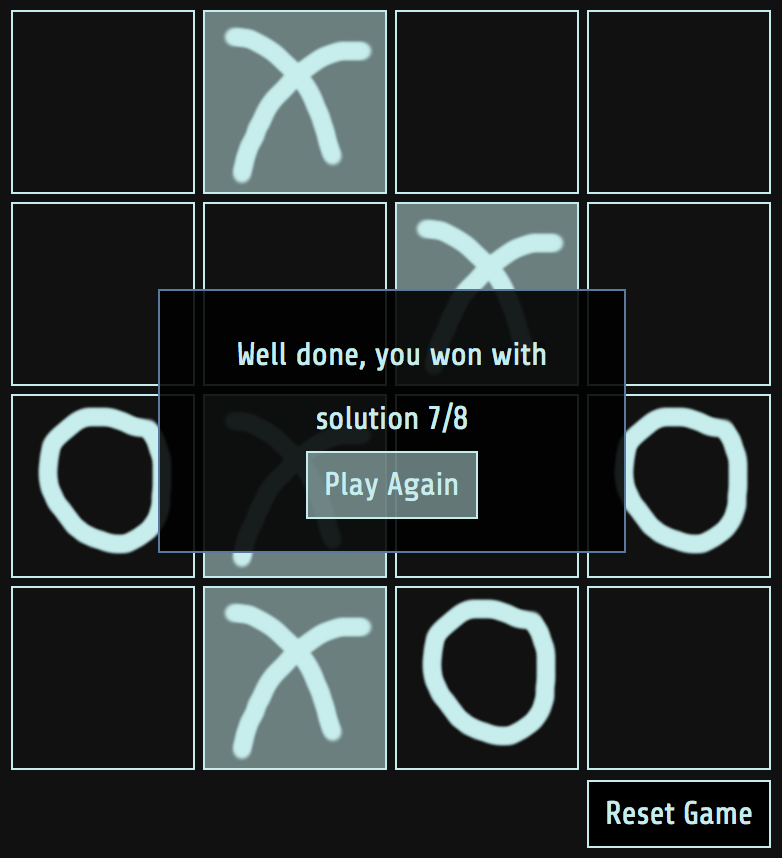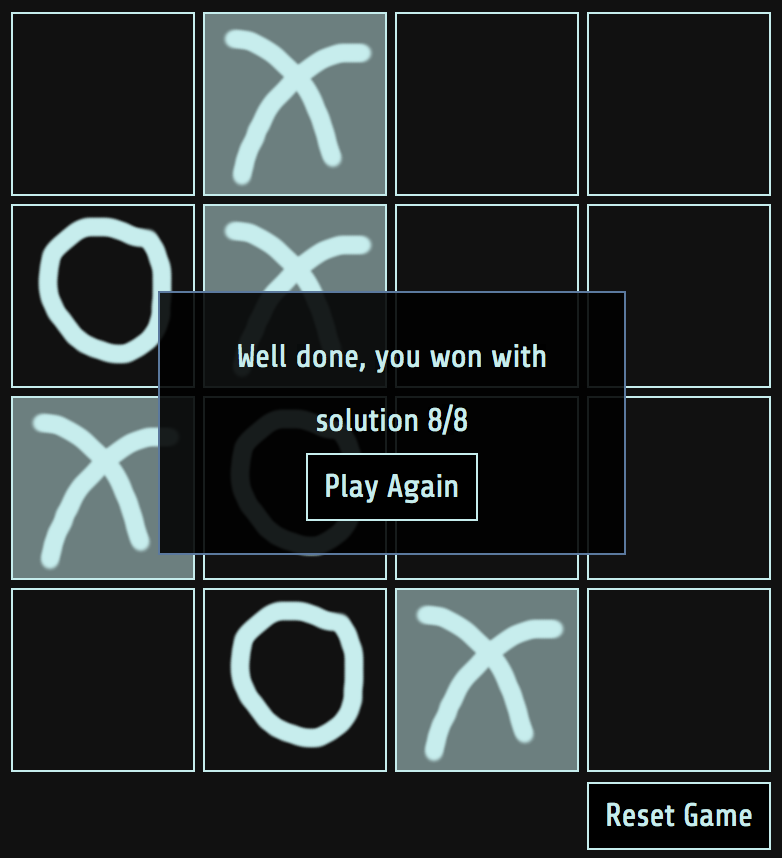We notice that in all these solutions there is exactly one X in each row. We can extract 2 bits from each row, and so each solution provides an entire byte. We can do this by labelling each column as 00, 01, 10 and 11 such that solution 1 corresponds to 01-00-01-11. Repeating for each solution, we get the binary message to be:

```01000111 01100001 01001101 00110011
00110000 01010110 01100101 01010010
```

Thus the solution, assuming ASCII encoding, is `GaM30VeR`.